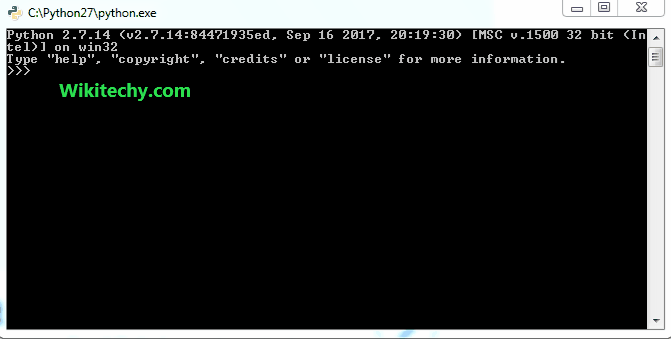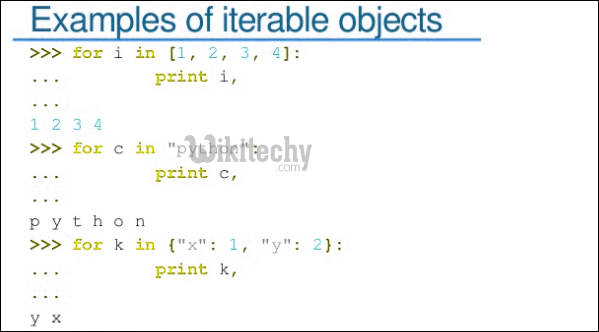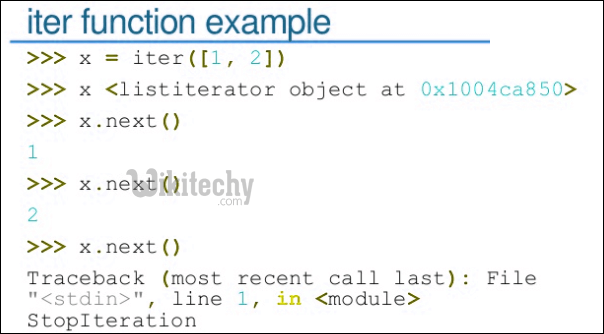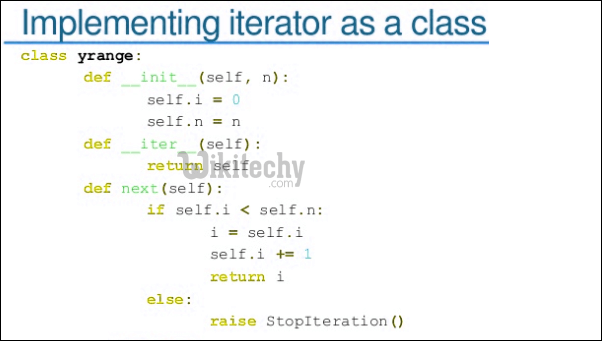# python tutorial - Iterator Python | Iterators in Python - learn python - python programmingLearn Python - Python tutorial - python iterator - Python examples - Python programs

Iterator in python is any python type that can be used with a ‘for in loop’. Python lists, tuples, dicts and sets are all examples of inbuilt iterators. These types are iterators because they implement following methods. In fact, any object that wants to be an iterator must implement following methods.1. __iter__ method that is called on initialization of an iterator. This should return an object that has a next or __next__ (in Python 3) method.
2. next ( __next__ in Python 3) The iterator next method should return the next value for the iterable. When an iterator is used with a ‘for in’ loop, the for loop implicitly calls next() on the iterator object. This method should raise a StopIteration to signal the end of the iteration.Below is a simple Python program that creates iterator type that iterates from 10 to given limit. For example, if limit is 15, then it prints 10 11 12 13 14 15. And if limit is 5, then it prints nothing.python - Sample - python code :

python tutorial - Output :

```10
11
12
13
14
15```

Examples of inbuilt iterator types.python - Sample - python code :

python tutorial - Output :

```List Iteration
wikitechy
best e-learning
website

Tuple Iteration
wikitechy
best e-learning
website

String Iteration
W
i
k
i
t
e
c
h
y

Dictionary Iteration
xyz  123
abc  345```

Wikitechy tutorial site provides you all the learn python , python programming help ,online python learning , learn to program with python , online python training free